# How can I solve these two physics problems? (equilibrium and moment)

• Tapias5000
In summary, the conversation discusses a problem with finding the correct solution for calculating the weight and torque of a force in a given scenario. The participants also discuss different methods and approaches for solving the problem, including using symbols and keeping everything symbolic until the final step. They also mention a mistake in the original equation and clarify the use of the sin and cos functions in determining the vertical component of the force. f

#### Tapias5000

Homework Statement
1) The non-elongated length of the spring AB is 8 m. If the block is held in the equilibrium position equilibrium position shown, determine the mass of the block at D.
2) Replace the system acting on the frame by an equivalent resultant force and specify the point, measured from B, where the line of action of the resultant intersects element BC.
Relevant Equations
1) Σfx=0, Σfy=0 and F=kx
2) Σfx=0, Σfy=0, Θ=arctan(Σfy/Σfx) and ΣMb=Mrb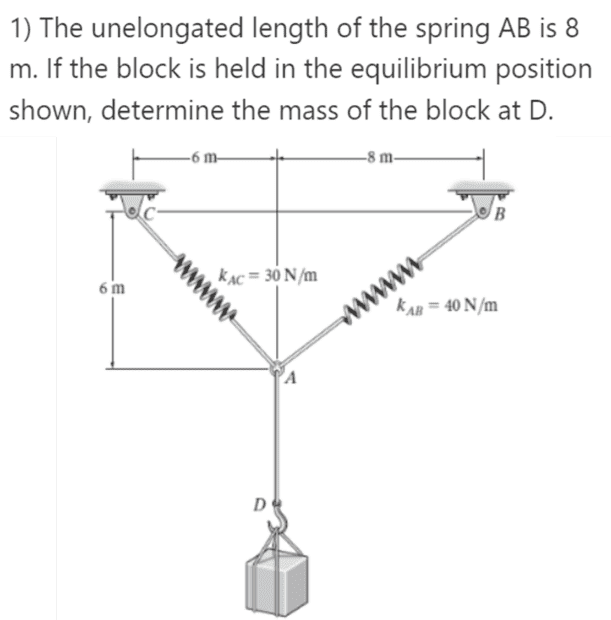I tried to solve it and I got the following is it correct?and 2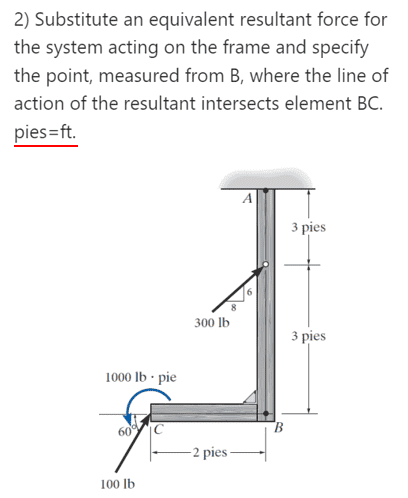My solution... is a negative distance?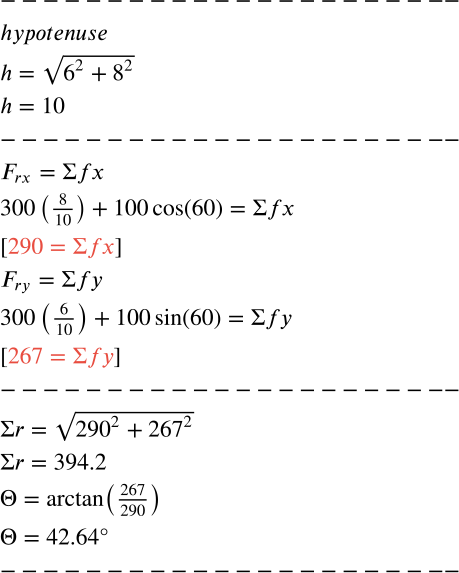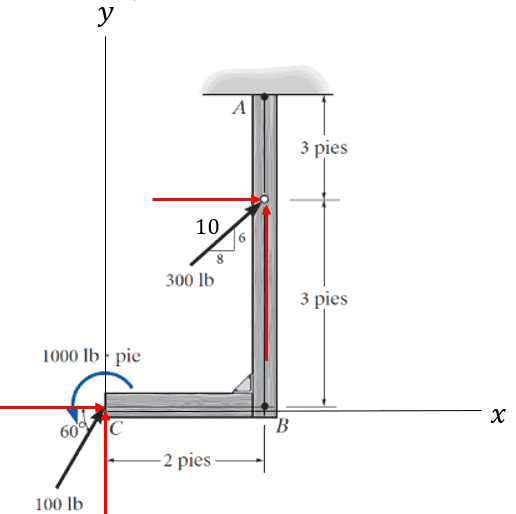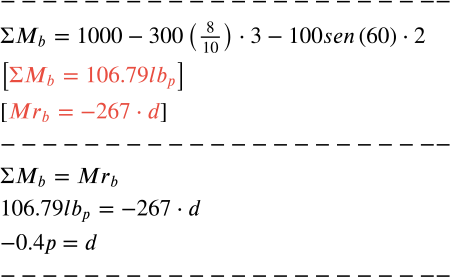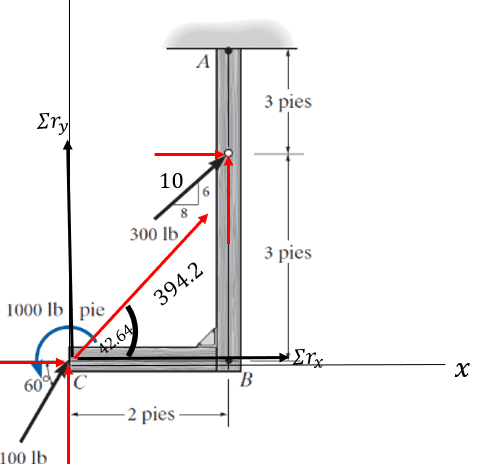•Delta2
1. The horizontal and vertical components of the force in AC must be equal, so you can write the weight equals ##F_{AB}(\frac 35+\frac 45)##.
2. Suppose the point is x to the left of B and write that the net torque about that point is zero:
##-\frac 453(300)+\frac 35x(300)+1000+\frac{\sqrt 3}22(100)(x-2)=0##.

Whoops, typo. Should be ##-\frac 453(300)+\frac 35x(300)+1000+\frac{\sqrt 3}2(100)(x-2)=0##

As a matter of general style, I strongly recommend keeping everything symbolic until the final step, creating symbols as necessary for given numerical values. It has many advantages, including, often, less arithmetic and greater precision.
Ok, I violated my own principle just above, but if you had created symbols for the forces and lengths I would have preferred to use them.

Last edited:
•Delta2 and Tapias5000
1. The horizontal and vertical components of the force in AC must be equal, so you can write the weight equals ##F_{AB}(\frac 35+\frac 45)##.
2. Suppose the point is x to the left of B and write that the net torque about that point is zero:
##-\frac 453(300)+\frac 35x(300)+1000+\frac{\sqrt 3}22(100)(x-2)=0##.

As a matter of general style, I strongly recommend keeping everything symbolic until the final step, creating symbols as necessary for given numerical values. It has many advantages, including, often, less arithmetic and greater precision.
Ok, I violated my own principle just above, but if you had created symbols for the forces and lengths I would have preferred to use them.
bruh, I can barely understand what I'm doing enough to apply other formulas... sad.

in 3/5*x*300 generates angular momentum at b?
also by doing your formula I get a different value at x and it is also positive.

in 3/5*x*300 generates angular momentum at b?
No, but we are interested in the moment about a point distance x to the left of B. Both horizontal and vertical components of the 300lb force can have a moment about that.
also by doing your formula I get a different value at x and it is also positive.
I made a typo in the LaTeX, doubling up a 2. See the corrected post.

•Tapias5000
No, but we are interested in the moment about a point distance x to the left of B. Both horizontal and vertical components of the 300lb force can have a moment about that.

I made a typo in the LaTeX, doubling up a 2. See the corrected post.
oooh, indeed the result is the same, is a negative distance logical?
how do you know that you can equal it to 0? do you have any video where you explain this method?
how do you get that sqrt(3)/2, and how do you know where to put x?

Sorry, this is the first time I see this method...

oooh, indeed the result is the same, is a negative distance logical?
how do you know that you can equal it to 0? do you have any video where you explain this method?
how do you get that sqrt(3)/2, and how do you know where to put x?

Sorry, this is the first time I see this method...
I understand that sin(60)=sqrt(3)/2
but cos(60)=1/2 how do you get a factor of sqrt(3)/2 then?

oooh, indeed the result is the same, is a negative distance logical?
how do you know that you can equal it to 0? do you have any video where you explain this method?
how do you get that sqrt(3)/2, and how do you know where to put x?

Sorry, this is the first time I see this method...
Suppose the equivalent force is F passing through the line BC at point P. The force has no moment about P, so the system of forces has no moment about P.

It certainly could turn out to be to the right of B. We know F's vertical component must be up the page, so the stronger the anticlockwise applied torque at C, the further to the right F must act.

•Tapias5000
I understand that sin(60)=sqrt(3)/2
but cos(60)=1/2 how do you get a factor of sqrt(3)/2 then?

It's the vertical component of the force at C. Since the horizontal component acts along BC, it can have no moment about P, but the vertical component will be distance x-2 to the left of P.

•Tapias5000
ooh okay, i think I get the idea

It's the vertical component of the force at C. Since the horizontal component acts along BC, it can have no moment about P, but the vertical component will be distance x-2 to the left of P.
thank you for the clarifications.

•Delta2